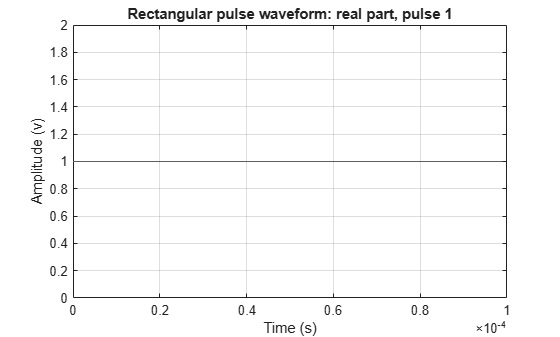plot

System object: phased.RectangularWaveform
Package: phased

Plot rectangular pulse waveform

Syntax

plot(Hwav)
plot(Hwav,Name,Value)
plot(Hwav,Name,Value,LineSpec)
h = plot(___)

Description

plot(Hwav) plots the real part of the waveform specified by Hwav.

plot(Hwav,Name,Value) plots the waveform with additional options specified by one or more Name,Value pair arguments.

plot(Hwav,Name,Value,LineSpec) specifies the same line color, line style, or marker options as are available in the MATLAB® plot function.

h = plot(___) returns the line handle in the figure.

Input Arguments

 Hwav Waveform object. This variable must be a scalar that represents a single waveform object. LineSpec Character vector to specifies the same line color, style, or marker options as are available in the MATLAB plot function. If you specify a PlotType value of 'complex', then LineSpec applies to both the real and imaginary subplots. Default: 'b'

Name-Value Arguments

Specify optional comma-separated pairs of Name,Value arguments. Name is the argument name and Value is the corresponding value. Name must appear inside quotes. You can specify several name and value pair arguments in any order as Name1,Value1,...,NameN,ValueN.

PlotType

Specifies whether the function plots the real part, imaginary part, or both parts of the waveform. Valid values are 'real', 'imag', and 'complex'.

Default: 'real'

PulseIdx

Index of the pulse to plot. This value must be a scalar.

Default: 1

FrequencyOffset

Frequency offset

Frequency offset in Hz, specified as a scalar.

Dependencies

This property applies when you set the FrequencyOffsetSource property to 'Input port'.

Default: 0 Hz

Output Arguments

 h Handle to the line or lines in the figure. For a PlotType value of 'complex', h is a column vector. The first and second elements of this vector are the handles to the lines in the real and imaginary subplots, respectively.

Examples

expand all

Create and plot a 100 μs rectangular pulse waveform.

waveform = phased.RectangularWaveform('PulseWidth',100e-6);
plot(waveform);